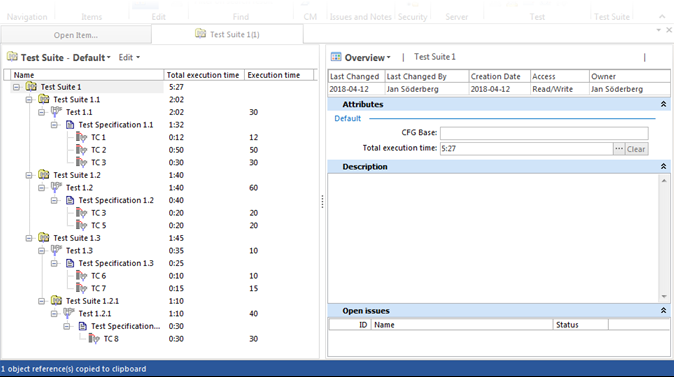If there is a need to manage test execution time, using an Integer attribute and a Computed attribute could be one solution.

In our example, the execution time is the time required for performing an individual Test Case and the set-up time required for the complete Test.

An 'Execution time' [5TCE] integer attribute is set as a default attribute on Test Case and Test with a scale of minutes. The value is stored on Test rather than Test Specification (which would make it easier to reuse the value, along with the Test Specification) because the set-up time depends on the test environment which is under the Test level. Using the same argument, the 'Execution time' ought to be a node/part attribute of the Test Case, under the Test (or maybe Test Specification) but this would make the solution a bit more difficult to manage, since such attributes would require editing using a special grid view.

The total of 'Execution time' is calculated by a Computed attribute 'Total execution time' [5TTE], which summarizes the time on Test Suite level (including all sub Test suits) and Tests/Test Cases:

`/ISSS*/ISES*/ISSP*/ITEC[@5TCE > 0].Select(@5TCE).Sum + /ISSS*/ISES*[@5TCE > 0].Select(@5TCE).Sum`

Note: The condition [@5TCE > 0] is required since the sum would otherwise potentially be calculated over 'nil' values for those attributes that have no value, which would result in a 'nil' sum. If the attribute is introduced before the first Test Case is created, this condition is not needed as the attribute would have a default value of 0 min.

An alternative method is to present the Total execution time in the h:m format:

`minutes := /ISSS*/ISES*/ISSP*/ITEC[@5TCE > 0].Select(@5TCE).Sum + /ISSS*/ISES*[@5TCE > 0].Select(@5TCE).Sum; hours := minutes.Div( 60); minute:= minutes - (hours * 60); hours.ToString + ":" + minute.ToString`

This method, however, displays 1 hour + 3 minutes as: "1:3".

The following method displays the time as "1:03":

`minutes := /ISSS*/ISES*/ISSP*/ITEC[@5TCE > 0].Select(@5TCE).Sum + /ISSS*/ISES*[@5TCE > 0].Select(@5TCE).Sum; hours := minutes.Div( 60); minute:= minutes - (hours * 60); textminute := if minute < 10 then "0" + minute.ToString else minute.ToString; hours.ToString + ":" + textminute`

# Result